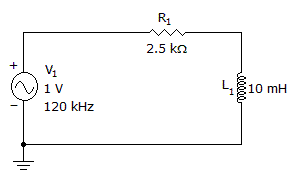# Electronics - Inductors

### Exercise :: Inductors - General Questions

43.

A practical inductor

 A. has inductance B. has winding resistance C. has winding capacitance D. all of the above

Answer: Option D

Explanation:

No answer description available for this question. Let us discuss.

44.

When current in an inductor starts to change, it cannot react instantly due to:

 A. fixed coil resistance B. eddy current generation C. applied emf of the circuit D. the counter emf of the coil

Answer: Option D

Explanation:

No answer description available for this question. Let us discuss.

45.

What is the approximate time for a complete decay of 400 mA of inductor current, when R = 10 ohms and L = 20 H?

 A. 10 seconds B. 8 seconds C. 4 seconds D. 2 seconds

Answer: Option A

Explanation:

No answer description available for this question. Let us discuss.

46.

A utility pole transformer used to supply a residential customer with two phases uses:

 A. two phases on the primary B. a center tap on the secondary C. a separate ground for each phase D. a small coefficient of coupling

Answer: Option B

Explanation:

No answer description available for this question. Let us discuss.

47.

What is the inductive reactance?A. 5.2B. 7.5C. 1.3 kD. 7.5 kAnswer: Option D

Explanation:

No answer description available for this question. Let us discuss.

48.

When a coil collapses the induced circuit current will:

 A. be in the same direction B. go to zero C. aid counter emf buildup D. be in the opposite direction

Answer: Option A

Explanation:

No answer description available for this question. Let us discuss.

49.

When a rate of one ampere per second causes an induced voltage of one volt, the unit of measure is

 A. a lenz B. an ohm C. a farad D. a henry

Answer: Option D

Explanation:

No answer description available for this question. Let us discuss.

#### Current Affairs 2021

Interview Questions and Answers